# Floyd: MCQ in Transistor Bias Circuits

(Last Updated On: December 5, 2019)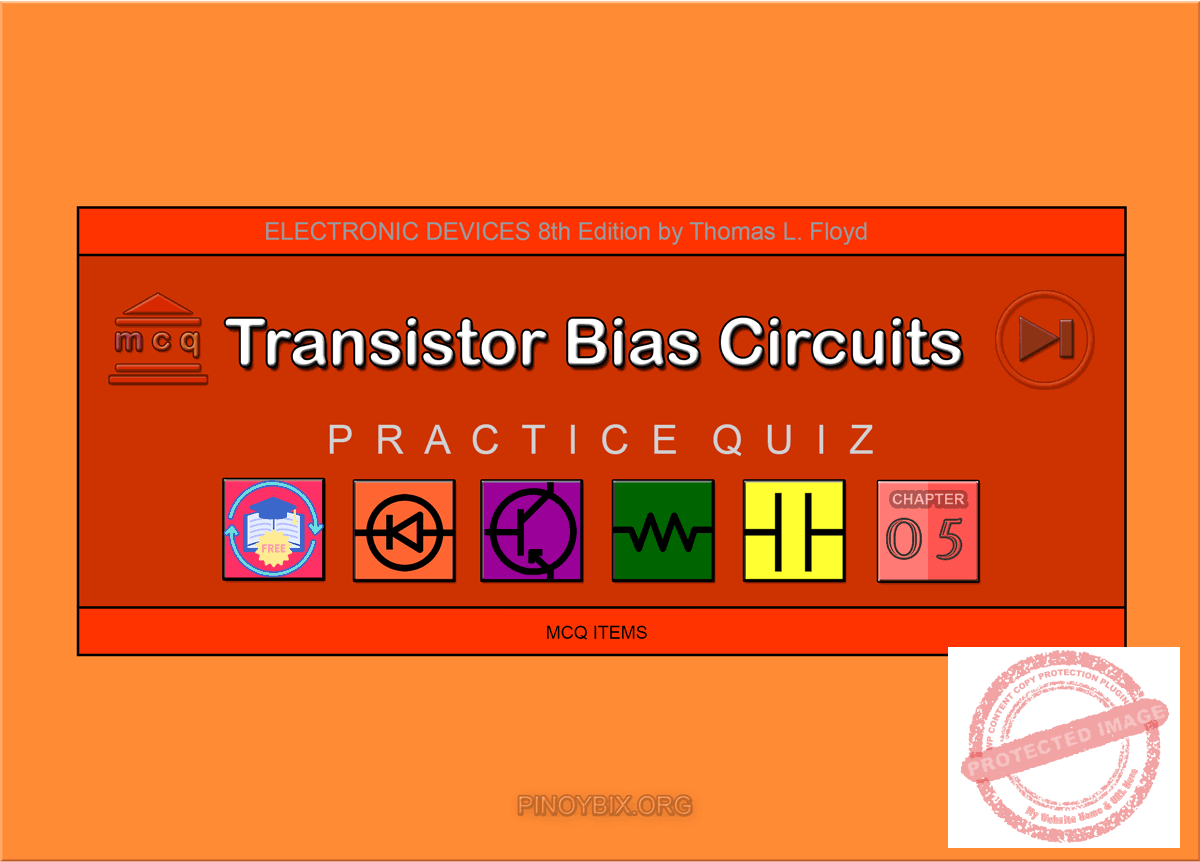This is the Multiple Choice Questions in Transistor Bias Circuits from the book Electronic Devices – Electron Flow Version and Conventional Current Version 8th Edition by Thomas L. Floyd. If you are looking for a reviewer in Electronics Engineering this will definitely help. I can assure you that this will be a great help in reviewing the book in preparation for your Board Exam. Make sure to familiarize each and every questions to increase the chance of passing the ECE Board Exam.

#### Online Questions and Answers Topic Outline

• MCQ in Transistor Bias Circuits
• MCQ in The DC Operating Point
• MCQ in Voltage-Divider Bias
• MCQ in Other Bias Methods
• MCQ in GreenTech Application 5: Wind Power

If you are looking for the Self-test in Floyd’s Electronic Devices proceed to

#### Practice Exam Test Questions

Choose the letter of the best answer in each questions.

1. Voltage-divider bias has a relatively stable Q-point, as does

A) base bias.

B) collector-feedback bias.

C) both of the above

D) none of the above

Solution:

2. Emitter bias requires

A) only a positive supply voltage.

B) only a negative supply voltage.

C) no supply voltage.

D) both positive and negative supply voltages.

Solution:

3. Clipping is the result of

A) the input signal being too large.

B) the transistor being driven into saturation.

C) the transistor being driven into cutoff.

D) all of the above

Solution:

4. Changes in βDC result in changes in

A) IC.

B) VCE.

C) the Q-point.

D) all of the above

Solution:

5. The input resistance at the base of a voltage-divider biased transistor can be neglected

A) at all times.

B) only if the base current is much smaller than the current through R2 (the lower bias resistor).

C) at no time.

D) only if the base current is much larger than the current through R2 (the lower bias resistor).

Solution:

6. What is the Q-point for a fixed-bias transistor with IB = 75 µA, βDC = 100, VCC = 20 V, and RC = 1.5 kΩ?

A) VC = 0 V

B) VC = 20 V

C) VC = 8.75 V

D) VC = 11.25 V

Solution:

7. Ideally, for linear operation, a transistor should be biased so that the Q-point is

A) near saturation.

B) near cutoff.

C) where IC is maximum.

D) halfway between cutoff and saturation.

Solution:

8. Refer to Figure 5-1. The value of IB is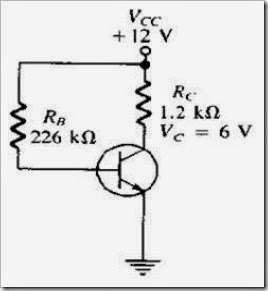Figure 5-1

A) 53 µA.

B) 50 µA.

C) 50 mA.

D) 53 mA.

Solution:

9. Refer to Figure 5-1. The value of IC is

A) 10 µA.

B) 10 mA.

C) 5 mA.

D) 50 mA.

Solution:

10. Refer to Figure 5-1. The value of βDC is

A) 5.3.

B) 53.

C) 94.

D) 100.

Solution:

11. Refer to Figure 5-2. Determine IC.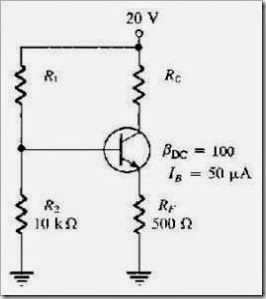Figure 5-2

A) 5 µA

B) 5 mA

C) 0 mA

D) 10 mA

Solution:

12. Refer to Figure 5-2. Assume that IC ≈ IE. Find VE.

A) 5 V

B) 10 V

C) 15 V

D) 2.5 V

Solution:

13. Refer to Figure 5-2. Assume IC ≈ IE. Determine the value of RC that will allow VCE to equal 10 V.

A) 1 kΩ

B) 1.5 kΩ

C) 2 kΩ

D) 2.5 kΩ

Solution:

14. Refer to Figure 5-2. Calculate the current I2.

A) 32 mA

B) 3.2 mA

C) 168 µA

D) 320 µA

Solution:

15. Refer to Figure 5-3(a). The most probable cause of trouble, if any, from these voltage measurements would be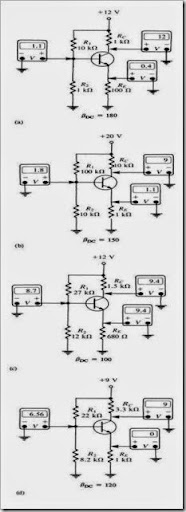Figure 5-3

A) the base-emitter junction is open.

B) RE is open.

C) a short from collector to emitter.

D) no problems.

Solution:

16. Refer to Figure 5-3(b). The most probable cause of trouble, if any, from these voltage measurements is

A) the base-emitter junction is open.

B) RE is open.

C) a short from collector to emitter.

D) no problems.

Solution:

17. Refer to Figure 5-3(c). The most probable cause of trouble, if any, from these voltage measurements is

A) the base-emitter junction is open.

B) RE is open.

C) a short from collector to emitter.

D) no problems.

Solution:

18. Refer to Figure 5-3(d). The most probable cause of trouble, if any, from these voltage measurements is

A) the base-emitter junction is open.

B) RE is open.

C) a short from collector to emitter.

D) no problems.

Solution:

19. The most stable biasing technique used is the

A) voltage-divider bias.

B) base bias.

C) emitter bias.

D) collector bias.

Solution:

20. At saturation the value of VCE is nearly _____, and IC = _____.

A) zero, zero

B) VCC, IC(sat)

C) zero, I(sat)

D) VCC, zero

Solution:

21. The linear (active) operating region of a transistor lies along the load line below _____ and above _____.

A) cutoff, saturation

B) saturation, cutoff

C) cutoff, cutoff

D) saturation, saturation

Solution:

22. What is the most common bias circuit?

A) base

B) collector

C) emitter

D) voltage-divider

Solution:

23. What is the dc input resistance at the base of a BJT?

A) βDCRC

B) βdc • (RC || RE)

C) βDC • re′

D) βDCRE

Solution:

24. Which transistor bias circuit provides good Q-point stability with a single-polarity supply voltage?

A) base bias

B) collector-feedback bias

C) voltage-divider bias

D) emitter bias

Solution:

25. Which transistor bias circuit arrangement has poor stability because its Q-point varies widely with βDC?

A) base bias

B) collector-feedback bias

C) voltage-divider bias

D) emitter bias

Solution:

26. Which transistor bias circuit arrangement provides good Q-point stability, but requires both positive and negative supply voltages?

A) base bias

B) collector-feedback bias

C) voltage-divider bias

D) emitter bias

Solution:

27. Which transistor bias circuit arrangement provides good stability using negative feedback from collector to base?

A) base bias

B) collector-feedback bias

C) voltage-divider bias

D) emitter bias

Solution:

28. Refer to Figure 5-4. In the voltage-divider biased npn transistor circuit, if RC opens, the transistor is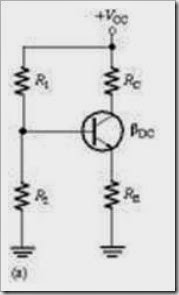FIGURE 5-4

A) saturated.

B) cutoff.

C) nonconducting.

D) none of the above

Solution:

29. Refer to Figure 5-4. In the voltage-divider biased npn transistor circuit, if R2 opens, the transistor is

A) saturated.

B) cutoff.

C) nonconducting.

D) none of the above

Solution:

30. Refer to Figure 5-4. In the voltage-divider biased npn transistor circuit, if R1 opens, the transistor is

A) saturated.

B) cutoff.

C) nonconducting.

D) none of the above

Solution:

### Complete List of Chapter Quiz in Floyd’s Electronic Devices

P inoyBIX educates thousands of reviewers and students a day in preparation for their board examinations. Also provides professionals with materials for their lectures and practice exams. Help me go forward with the same spirit.

“Will you subscribe today via YOUTUBE?”

Subscribe

PinoyBIX Engineering. © 2014-2020 All Rights Reserved | How to Donate? |